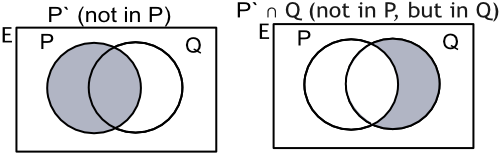Manipulating Sets

## Manipulating Sets

3 ∈ {odd numbers} means that 3 is an element of the set of odd numbers.

2 ∉ {odd numbers} means that 2 does not belong to that set.

A subset is a set where elements in the subset are part of another set. A subset cannot include elements that are not in the original set.

If only some of the elements of a subset appear in the original set, then that is called a proper subset, and will have fewer elements than the original set. A proper subset is shown as D ⊂ E, where D is a proper subset of E. If it is not a proper subset, then the symbol has a diagonal line through it.

A subset of data that matches the original set is shown as B ⊆ A (B is a subset of or equal to A).

Set Pc (sometimes P ) are all the elements that are not in P: this is the complement of set P.An empty set is shown with the sign ∅, and represents the set { }.

## Example 1

The number cards in a suit of playing cards are defined as the universal set, so

xi = {2, 3, 4, 5, 6, 7, 8, 9 and 10}.

If E contains the set of even numbers, write the members of Ec.

The complement of E is required: those elements that are not in E. Use a small c to show it is a complement.

Answer: Ec = {3, 5, 7, 9}

## Example 2

Number cards in a single suit of playing cards are defined as the universal set:

xi = {2, 3, 4, 5, 6, 7, 8, 9 and 10}.

If E contains the set of even numbers, and T contains the set of numbers that are a multiple of 3, write the set for E^c uu T.

E = {2, 4, 6, 8, 10}

The complement of E is required, elements in the universal set that are not in E:

E^c = {3, 5, 7, 9} and T = {3, 6, 9}

The union is required, elements in both lists: E^c uu T = {3, 5, 6, 7, 9}

Answer: E^c uu T = {3, 5, 6, 7, 9}`